## Visual Numbers: The Next Generation (I)

Hey, just because I’m an English major now doesn’t mean I can’t enjoy a bit of math on the side. Therefore, I present to you Visual Numbers: The Next Generation (Part I, insert obligatory Star Wars joke).

I’ve always been a big fan of fractals. I was imagining fractal-esque recursive structures back when solving 2x + 5 = 0 seemed intimidating. Recently, in my wanderings through the hallowed halls of physics, I stumbled across the idea of generating fractals by coloring each pixel of an image according to the final state of a differential equation, with the x and y coordinates of that pixel as input parameters. Of course, being an incompetent programmer, I haven’t been able to write a stable integrator for a differential equation, but I’m just smart enough to manage simple stuff like the discrete logistic map, where every new x is computed according to the formula x = λx(1-x), where λ is a constant. The other day, I had a brainstorm and decided to create a two-dimensional version of the same sort of thing, only with x as a vector and replacing x at each step with F(x), where F is a vector function. Basically, all that means is that I now have two integer variables to play with, which allows for more interesting behavior and allows me to create pretty pictures like this:In this image, each pixel is colored according to the following rule: all pixels start out white. Then, each pixel is turned into a vector v(x,y), where x and y are the pixel’s coordinates. The function v(x,y) = v(-x.y,x.x + floor(0.5 * x.y)) is then applied repeatedly for a fixed number of steps (in this case, 750). If at any point during iteration the vector reaches a previously-visited point, then that point forms a closed, repetitive orbit in 2-space and the pixel is colored black. If this doesn’t happen, the pixel is left white.

The shapes here are pretty nice, even if I do say so myself. From some prior experiments with similar mappings, I think that the smaller black ellipses with banding and satellite blobs represent orbits shaped like three elliptical orbits connected into a weird triangle, and that the large oval with the large banding represents the “spirograph-like” orbits.

The really neat thing about mappings like this is that they’re a (relatively) computationally-inexpensive alternative to the differential-equation mappings I discussed earlier. I’m no great mathematician (obviously), but I get the feeling that these two-dimensional mappings are discretized analogues of the mapping that generates the ever-beautiful Mandelbrot Set.

Interestingly (and here I’m playing mathematician again), you can write this type of mapping this way:

F(v) = v(ax + by,cx + dy). In the case of the above map, a = 0, b = -1 , c = 1, d = 0.5.  With a = 0.5, b = -1, c = 1, and d = 0.1, you get a beautiful spiral pattern:It’s a little hard to see because the orbits are so dense, but this pattern is actually fractal, too (or seems to be): there are smaller spirals to the top-center and left-center of the big one, and what looks like unformed proto-spirals in between those.And this lovely pattern is created by a = 0, b = -1, c = 1, d = 0.1. Note the eleven-pointed stars in the upper right and left and the lower right. Watch this space for more mathematical prettiness.

## Visual Numbers #3

It’s been nine months since Visual Numbers #1 and #2, and now, thanks to one sleep-deprived evening of daydreaming at my parents’ house, I bring you #3. Sorry about the images’ weird dimensions, but the reason for those dimensions will become clear.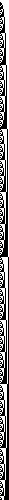Binary Progression: The numbers from 1 to 500, converted into binary and drawn as black and white squares. Each number is a series of 1s and 0s, and the 1s are drawn as black squares and the 0s as white squares.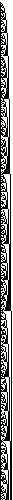Binary Primes: The prime numbers between 1 and 5000 (I think), graphed in the same way as above. I was kind of disappointed by this, honestly. I was kind of hoping all the squares would spell out “CONGRATULATIONS, YOU FOUND A PATTERN IN THE PRIMES” or something.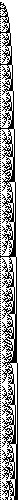Binary Squares: The squares of every number between 1 and 500. Note the interesting fractal pattern.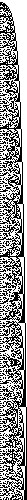Binary Cubes: The numbers from 1 to 500, cubed and displayed in binary. Notice how this one looks a lot more chaotic than did the last one.

## Visual Numbers #2Factors: The numbers from 1 to 500 are plotted horizontally across the top row. Along each vertical column, if N divides the number X (represented here by distance across the top row) evenly (that is, if N is a factor of X), then the pixel N pixels down from the top is black.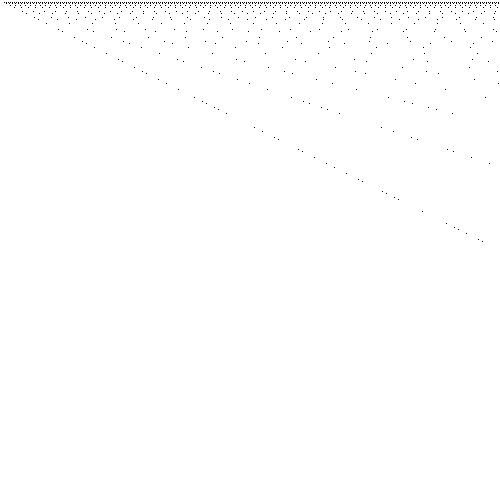Prime Factors: The same general principle as above, but in this image, only the prime factors are shown.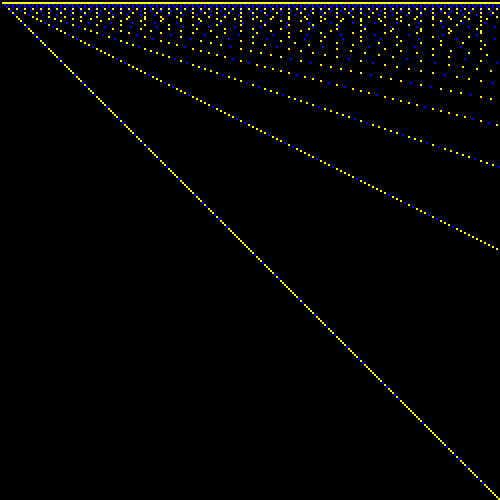Blue Over Yellow: Basically, a combination of the previous two images. Numbers from 1 to 250 are plotted horizontally, and factors are plotted vertically. If a factor is prime, the little square representing it is blue, otherwise, it’s yellow.

That tantalizing structure is still just slightly out of reach…Oh well, back to work!

## Visual Numbers #1

This is the beginning of what I hope will be a fairly long-running series of posts, each containing one or two (or three, if I’m feeling adventurous) numerical or mathematical visualizations. If you need a concrete example of what I’m talking about, just check out the image here).

Anyway, here goes:Meet the Primes: Every pixel represents a number from 1 to 250,000. The image wraps horizontally; that is, the first pixel of the first row is the number 1, the first pixel of the second row is 501 (since each row is 500 pixels wide), the first pixel of the third row is 101, and so on and so on. Pixels representing prime numbers are black. From this view, it’s quite obvious that there’s likely some sort of structure to the primes, but it’s hard to say what that structure might be.

## A Labor of Love

A few days ago, I was mucking about in the vast swampland that the Internet has become, and I stumbled upon yet another reference to a programming language I’d heard about a few times before: “Processing.” My interest piqued, I went in search of a compiler, and found one at Processing’s website (www.processing.org), and immediately started learning the language.

Given my many previous failures trying to learn Java, upon which Processing is based, I didn’t think I’d have much chance of learning the language, but I tried anyway, and actually found it just about as intuitive and elegant as Python, which remains my favorite programming language of all times. For a while, I cobbled together various tiny programs to do things like graph functions in one and two variables, graph parametric equations by replacing x, y, and z with rgb color values (producing a rather strange-looking wave of color that wasn’t nearly as interesting as I’d hoped), and visualizing one-dimensional celluar automata (which, by the way, was a complete failure, because Python is the only language I’ve found whose array-handling I can both tolerate and understand). Then, since I’m always a mathematician at heart, I thought I’d do something that mathematicians love to do: visualize the primes.

Before I go on, I should re-iterate just how much of a godsend Processing is. I’ve been trying to write methods in Python to visualize various kinds of functions and data for many moons, and my results have never been much more than mediocre. The only graphics module I’ve learned in Python (Tkinter, in case you were interested) is clumsy and runs slowly, and really isn’t meant to handle the kind of pixel-by-pixel manipulations I’d had in mind. Processing, though, exists solely for this purpose, which is the reason for my gushing for the last three paragraphs.

Anyway, the primes. I put together a simple program that computes the gap between the current prime number and the last prime number (using the standard Sieve of Eratosthenes method), and draws a circle at the location of the current prime whose size is based on the gap between the two primes.

I suspect I could have saved these thousand words by doing as the cliché says, and just giving you the picture:(You can see a higher-resolution version here).

There’s a great deal of hidden beauty in this picture, most of which I can’t claim responsibility for. There’s a certain order to it, even though the primes seem to be quite random. Really, the beauty comes from the delicate, elegant structure of mathematics. The structure of the primes, as the structure of pi, is an expression of the deep structure of numbers, and thus, of the deep structure of the universe itself. It can be an almost religious experience, a sort of holy communion with the Numbers, to be given a glimpse of that structure.

I don’t know why I’ve been so sentimental lately…either way, the point I’m driving at is this: visualization is a really powerful tool for understanding mathematics. And Processing is a great programming language for visualization. (And, once again, I sound like I’m on somebody’s payroll, but I’m not. As far as you know.).

## Political Sum-Over-Histories (and a solemn note on the Virginia Tech shootings)

Note: Being a reasonably decent human being, I feel I would be terribly remiss if I did not give my heartfelt condolences to the faculty and staff of Virginia Tech, following yesterday’s terrifying shooting. As a member of the college-going population, I myself was absolutely horrified that such a thing is even possible. As for the killer, who may have fancied that he would render the authorities powerless by killing himself before they could, I say they drag his rotten corpse behind a dump truck for a while. Now, please don’t fault me on this, dear readers, but I feel that the best course of action for me would be to simply go on as normal, as nothing I can do will change the facts.

## The Complete Guide to Ultrafunctions

Okay, I promise, this is going to be the last post I write about ultrafunctions, but I just wanted to gather all the information on them in one place.

Definition 1: The ultrafunction$u_n\left(f\left(x\right)\right)$ is defined as$f\left(f\left(\dots f$$a$$\dots\)$ (applied b) times

Theorem 1: f’s range must be the same set as, or a subset of, its domain (that is,$f:A\rightarrowA$)

Proof: Consider$f(f(a))$. Since f is a function in a single argument a, it only makes sense to apply f to itself if f’s result is contained in its domain. This can naturally, and easily be extended to a larger chain of functions [such as$f(f(f(a)))$]. Q.E.D.

## A Theory of Theories

The other day, I was having a conversation with a friend of mine, and I got thinking about scientific theories. I was maily considering the purported “theories of everything” (string theory, loop quantum gravity, twistor theory, etc.), and I began to wonder: has anyone developed or attempted to develop a “theory of theories of everything”? Would such an enterprise be fruitful or a waste of time?

Well, from this station, my train of thought kind of lost its brakes and went speeding down the hill…and I got wondering yet again: is it possible that no such theory of everything exists? Is it possible that humans will get caught in an unending series of revisions, each theory matching nature more and more closely, but each theory still having its own flaws and inaccuracies. But what about the multiverse? Even if there is no “theory of everything” in our universe, might there not be one in a different universe? What this question boils down to is this: Is it possible that the underlying mathematical and theoretical structure of a particular bit of the multiverse universal, or is it local? Might mathematics be more or less consistent (removing or worsening things like Russell’s paradox and Gödel’s incompleteness problem) in other universes?

Just some more food for thought…

## Ultrafunctions and Transformations

I know I’ve written a whole ream of entries on this subject, but as I said before, it’s proved pretty darned fruitful.

Remember that the ultrafunction is defined thus:

u(f(a),b) = f(f(f(…f(a)…) (applied b times)

Now, consider the transformation t(g(a)) from the set of all functions into the set of all functions. Then, consider the ultrafunction of the transformation of the function:

u(t(f(a)),b) = t(f(t(f(t(f(…t(f(a))…) (as before, b repeats)

Would it be possible to “factor out” the transformation? Well, if that were possible, then we’d have:

t(f(f(f(…f(a)…) = t(f(t(f(t(f(…t(f(a))…)

But, since the transformations of two functions are equal only if they are taken of the same function, then:

f(f(f(…f(a)…) = f(t(f(t(f(t(…t(f(a))…)

Which will obviously only be true for a relatively small subset of the set of all functions.

## Yet More on the Ultrafunction

The ultrafunction really seems to be something of a mathematical gold mine (or at least a copper mine), and as I was thinking about it earlier, I’ve had some more ideas about it.

Recall that the ultrafunction is defined thus:

u(f(a),b) = f(f(f(…f(a)…) (repeated b times)

Are there any functions f_i(a) such that u(f_i(a),n) = f_i(a)? I shall refer to these as ultraidentity functions. Here are a few, defined on the set of real numbers:

f(x) = x

f(x) = c

There are also some semiultraidentity functions, which I’ll refer to as n-SI functions. The simplest example of a 2-SI function is:

f(x) = 1/x

Since 1/(1/x) = x, and then f(x) = 1/x, and so on ad infinitum.

f(x) = 1/(x^2), however, is not an ultraidentity function or an SI function, and in fact, taking the ultrafunction of 1/(x^2) repeatedly yields:

1/(1/x^2)^2 = x^4, 1/(x^4)^2 = 1/(x^8), etc.

## Ultrafunctions Revisited

I was contemplating the properties of the ultrafuncton (see the previous post), and I was wondering if there might be some higher-level version of the ultrafunction, and I realized that the ultrafunction itself is that function. For example:

u(f(a), n) = f(f(f(f(…a…))) (f applied n times)

But then, it follows naturally that

u(u(f(a), m), n) = u(u(u(…f(a)…), m), m) (ultrafunction applied n times)

So therefore, even though in many nested-function-type situations, there is an infinite hierarchy of such functions (consider Knuth’s up-arrow system), in this case, this single function fills in the hierarchy all by itself.

## Superfunctions and Ultrafunctions

Okay, so my first mathematics-related post was a bit of a letdown…but I think I’ve recovered some of my mathematical street cred (yes, mathematicians have street cred; how do you think they get into all the elite nightclubs?).

Suppose you have a function f(a, b) . I then define the “superfunction” s(f) of f(a, b) as:

s(f(a,b)) = f(a, f(a, f(a, … a))) (repeated b times)

Thus, s(a+b) = a * b, and s(a * b) = a ^ b, et cetera.

Consider also the “ultrafunction” u(f(a, b), c)of f(a, b) (of which the superfunction is a special case where c = 1; see below) thus:

u(f(a, b), c) = s(s(s(…f(a,b)…))) (c repeats)

u(a+b, 1) = a * b

u(a+b, 2) = a ^ b

s(f(a,b)) = u(f(a,b), 1)

## “I’m a math major, and thus unlikely to ever come to my senses.”

As I perused some of the other WordPress bloggers’ blogs, I began to realize a fundamental quality that my own blog lacked: content. One would expect that I would have realized this sooner, but apparently I was blinded by my lust for unending, nonsensical rants. But once I’d seen the light, that got me wondering; “What the hell have I got that’s worth talking about.” After several hours and many false starts, I realized I had at least one thing: mathematics.

Now, I do admit that I’m something of an outsider, even in mathematical circles. I’ve never been incredibly fond of the globs of terminology my fellow math-heads throw around (but that may be my ego doing the talking). Still, I think there’s something to be gained through a complete ignorance of all these extra words. (But I may be wrong: You are talking to a guy who didn’t take algebra until 9th grade, and hated math until he was about fifteen.)

Now, for those of you who slogged through all of that, here comes the mathematics:

NOTE: The moment I finished this article, I ran across the article on Wikipedia about Donald Knuth’s “Up-Arrow Notation.” It’s pretty much exactly the same as my n-multiplications. (I can hear all you mathematicians snickering at me…)

Sometime last semester, I got thinking about the different kinds of multiplication-like operations that could be performed, and I came up with a list of the “fundamental multiplications” (I now call them n-multiplications):

m_0(a, b) = 1

m_1(a, b) = a + b

m_2(a, b) = a * b

m_3(a, b) = a ^ b

I found the notebook I’d written this list in the other day, and it got me thinking: wouldn’t it be possible to write the higher-order multiplications in terms of the lower-order ones:

m_2(a, b) = a * b = a + a + a + … + a (b additions) = m_1(a, m_1(a, m_1(a, … a))) (b repeats)

And furthermore, you could write m3(a, b) thus:

m_3(a, b) = a ^ b = a * a * a * … * a (b multiplications) = m_2(a, m_2(a, m_2(a, … a)))

So, I took a bit of a leap, and in order to extend the idea of multiplications, I define the “property of reduction” for any multiplication m_n(a, b):

m_n(a, b) = m_(n-1) (a, m_(n-1)(a, … a)))

That’s about as far as it’s gone so far. If you really feel the need to correct me or add to the idea, e-mail the idea to heuristic000x@yahoo.com.

And yes, I’m aware that not all the n-multiplications are symmetric or associative. That’s one of those bits that remains to be added.